Discovering JMP > Work with Your Data > Work with Data Tables > Example of Calculating Values with Formulas
Publication date: 04/12/2021

## Example of Calculating Values with Formulas

Use the Formula Editor to create columns that contain calculated values.

### Scenario

The sample data table On-Time Arrivals.jmp reflects the percent of on-time arrivals for several airlines. The data was collected for March, June, and August of 1999.

### Create the Formula

Suppose that you want to create a new column containing the average on-time percentage for each airline.

1. Add a new column.

2. Right-click the column heading of the new column and select Formula. The Formula Editor window appears.

Figure 3.12 Formula Editor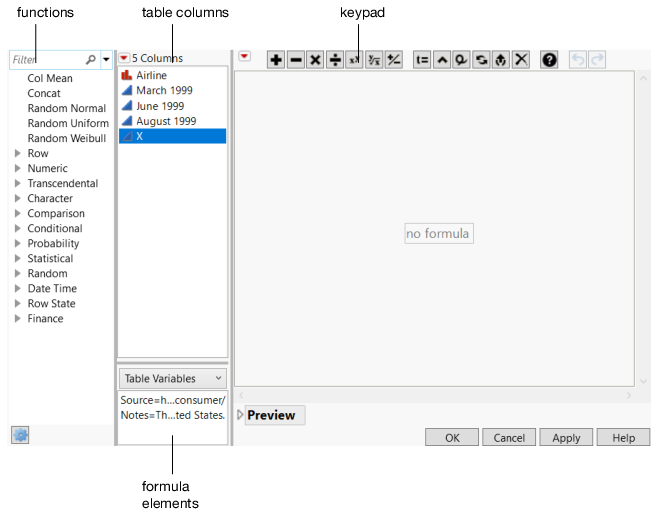Create the formula for the average on-time percentage of each airline:

3. From the Columns list, select March 1999.

4. Click the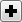button on the keypad.

5. Select June 1999, followed by anothersign.

6. Select August 1999.

Figure 3.13 Sum of the Months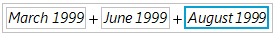Notice that only August 1999 is selected (has the blue box around it).

7. Click the box surrounding the entire formula.

Figure 3.14 Entire Formula Selected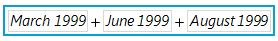8. Click the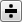button.

9. Type a 3 in the denominator box, and then click outside of the formula in any of the white space.

Figure 3.15 Completed Formula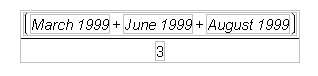10. Click OK

The new column contains the averages.

The Formula Editor has many built-in arithmetic and statistical functions. For example, another way to calculate the average on-time arrival percentage is to use the Mean function in the Statistical functions list. For more information about all of the Formula Editor functions, see Create Formulas in JMP in Using JMP.

Want more information? Have questions? Get answers in the JMP User Community (community.jmp.com).